# Investigation and Comparison of Fluoride Adsorption Behavior on a Hybrid Material Containing Zirconium Dioxide Coated on γ-Alumina (60 and 90) and Their Initial Precursor in Aqueous Solution

Document Type : Original Research Paper

Author

Material and Nuclear Fuel Scholl, Nuclear Science and Technology Research Institute, (NSTRI), Tehran, Iran

Abstract

Keywords

#### Full Text

INTRODUCTION

EXPERIMENTAL

Chemical and solutions

All the chemicals and reagents used in these experiments were of the analytical grade obtained from E. Merck or Fluka companies. Granular aluminum oxide-60 and 90 with the particle size of 0.063-0.2 mm (70-230 mesh) and different densities of 4 and 3.94 g.cm-3, respectively, were used in this research work. A stock solution of fluoride (1000 ppm) was prepared by dissolving sodium fluoride in demineralized water (DMW). The solution was further diluted to the required concentrations (5-100 ppm). To adjust the solution pH, nitric acid and sodium hydroxide solutions with a concentration of 0.1 mol.L−1 were used.

Preparation of zirconium oxide

Zirconium oxide nanoparticle was prepared via a sol-gel technique. Firstly, the proper amount of ZrOCl2·8H2O was added to 100 mL demineralized water with effective stirring (0.5 M ZrOCl2_8H2O). Afterward, to prepare zirconium hydroxide’s precipitation(Zr(OH)4), a little volume of 1M NaOH solution was gradually added to the above aqueous solution within 30 minutes in order to increase the pH value of the solution to 6.0-6.5 while the solution was continuously stirred at room temperature for 2 h. The formed precipitates were centrifuged and washed with distilled water several times. The obtained powder sample is finally dried overnight at 100 °C. The chemical reactions that occur are shown as follows.

Preparation of Al2O3-60-ZrO2 and Al2O3-90-ZrO2

To prepare Al2O3-60-ZrO2 and Al2O3-90-ZrO2 adsorbents, a solution of 0.1M containing zirconium oxychloride as the zirconium precursor source was used. The preparation involved the following steps: first, 4g of each of Al2O3-60 and Al2O3-90 was added separately to 600 mL of 0,1M zirconium oxychloride solution. These solutions were kept under constant stirring at room temperature for 2h. The materials were then separated by filtration and dried overnight in an oven at 100 °C.

Apparatus

The Brunauer-Emmett-Teller specific surface area, pore specific volume and pore diameter of the samples were measured by nitrogen adsorption/desorption at 77.4K using a Quantachrome nova station gas sorption analyzer. The XRD studies were made with a Philips model PW 1130/90. The quantitative determination of fluoride ions was carried out using fluoride selective electrode model ISTAK FOO1602. Waterbath shaker (model CH-4311, infors AG) was used throughout the work. The pH values of solution were measured with a Schott CG841 pH-meter (Germany).

To study fluoride adsorption behavior on zirconium oxide, Al2O3-60, Al2O3-90 and zirconium oxide embedded on Al2O3-60 and Al2O3-90, the batch equilibrium method was applied. The adsorption capacities (qt) and the percentage of fluoride adsorption (p) were determined according to the following equations, respectively.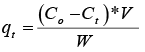(1)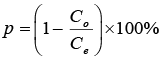(2)

where qt is the number of fluoride ions adsorbed onto adsorbent (mg.g-1) at time t; Co is the concentration of initial fluoride ions (ppm); Ct is fluoride ions concentration after a certain period of time (ppm); V is the initial solution volume (L); and m is the adsorbent mass (g).

In order to study the effect of initial fluoride concentration on the adsorption of fluoride, 100 cm3 polyethylene bottles containing 100 mg of each of the adsorbents and 20 cm3 of fluoride solution with the initial concentration (Cο) from 5 to 200 ppm and pH=5.5 were used. The bottles were capped and stored in a shaker operating at 298 K. The time of 24 hours and the stirring rate of 150 rpm was chosen to assure that the adsorption of fluoride was done completely. In addition, the effect of temperature on fluoride adsorption was studied at 288, 298, 308 and 318 K in the above-mentioned condition.

To investigate the effect of the solution pH value on fluoride adsorption at an initial concentration of 10 ppm, the number of hydronium ions in solution was adjusted at 10-3, 10-4, 10-5 and 10-6 M by adding negligible amount of concentrated nitric acid. Other conditions such as temperature, stripping rate and time were the same as above.

To study the effect of adsorbents mass on fluoride adsorption, the experiments were carried out by adding 25, 50, 75, 100, 125 and 150 mg of adsorbents into 20 mL of solution with pH=5.5 and initial fluoride concentration of 10 ppm.

Also, to study the effects of other anions such as sulfate and nitrate ions on fluoride adsorption, 20 mL fluoride solution containing an initial concentration of 10 ppm was mixed by 100 mg of adsorbents. The concentration of each of two anions (sulfate and nitrate ions) in the solution was adjusted at 0.001, 0.01, 0.1 and 1 M by adding a certain amount of sodium nitrate and sodium sulfate salts.

Finally, for the determination of equilibrium time, the times of 0.25, 0.5, 0.75, 1, 2, 5, 8 and 24 hours were selected and tested in the experimental conditions similar to the investigation of the effect of initial fluoride concentration on the adsorption of fluoride.

At the end of each test and after the separation of solids from the liquid phase by filtration through 0.45 µm membrane filters, the concentration of fluoride ions before and after equilibration was measured by ion-selective electrode method.

RESULT AND DISCUSSION

Characterization of synthesized materials

Fig. 1a and 1b exhibit N2 adsorption/desorption isotherm curves of Al2O3 (60 and 90) and ZrO2 embedded on Al2O3-60 and Al2O3-90, respectively. As can be seen, Al2O3-60 and Al2O3-90 samples show similar nitrogen absorption/desorption behavior while the typical isotherms of the Al2O3-60-ZrO2, Al2O3-90-ZrO2, and ZrOsamples are the same and show similar nitrogen absorption/desorption behavior, too. According to the original International Union of Pure and Applied Chemistry (IUPAC) classification, the convex plot to the relative pressure axis showed in Fig. 1a is characteristic of weak adsorbate-adsorbent interactions and it is most commonly associated with microporous adsorbents . However, isotherms with H3 type hysteresis loops which depicted for all of adsorbents in Fig. 1b (Al2O3-60-ZrO2, Al2O3-90-ZrO2, and ZrO2), are common features of type IV isotherms. This is commonly associated with the presence of mesoporosity structure in these adsorbents . Therefore, the structure of microporous in Al2O3-60 and Al2O3-90 and mesoporosity structure in Al2O3-60-ZrO2, Al2O3-90-ZrO2, and ZrOsamples were confirmed by this method.

The important physical properties such as specific surface areas and pore size distribution (including average pore diameter and pore volume) can be calculated using obtained N2 adsorption/desorption data, BET (Brunauer, Emmett, and Teller) and BJH (Barrett, Johner, and Halenda) equations. The calculated parameters are shown in Table 1. As can be seen, the BET surface area of Al2O3-60 and 90 samples decreased after loading ZrO2 onto them. This change can be due to the occupation of the Al2O3-60 and 90 microporous by the ZrO2. In addition, we can observe that the pore diameter of Al2O3-60 and Al2O3-90 decreases after the loading of ZrO2. This intimates that ZrO2 particles are mostly positioned on the external surface pore of these new adsorbents and confirm the immobilization of ZrO2 particles on Al2O3-60 and 90.

Crystalline structure and purity of the synthesized materials powder can be confirmed by powder X-ray diffractometer. The XRD patterns of Al2O3–ZrO2 and its pristine material has been shown in Fig. 2. As can be seen, the characteristic and diffraction peaks of Al2O3 (20.09°, 23.48°, 41.69*°, 43.24°, 67.2°) and ZrO2 (28.23°, 30.23°, 31.38°, 34.2°, 50.41°, 60.3°) match well with those of Al2O3 (Ref. code: JCPDS-00-013-0373) and ZrO2 (Ref. code: JCPDS-01-088-1007), respectively, which diffraction intensity of Al2O3 and ZrO2 peaks are become weaker and might be covered up in the XRD pattern of Al2O3–ZrO2 shown in Fig. 2. This confirms the successful immobilization of ZrO2 on Al2O.

Al2O3-60-ZrO2 ˃ Al2O3-60 ˃ Al2O3-90-ZrO2 ˃ Al2O3-90 ˃ ZrO2­­

As shown in Fig. 4, the adsorption percentage of fluoride on these adsorbents decreases with increasing fluoride concentration. This phenomenon can be referred to the occupation of constant adsorption active site on these adsorbents by the fluoride ions at low concentration. Therefore, there will not exist the available site to adsorption of fluoride at higher concentrations which consequently gives rise to decrease the adsorption percentage of fluoride.

The adsorption equilibrium data of fluoride on these adsorbents were analyzed by the mathematical-statistical models such as Langmuir, Freundlich, and Dubinin–Radushkevich (D–R) equations which describe the distribution of the adsorbate species between two phases, liquid and solid phase, at equilibrium condition. These models have been established on a set of assumptions which are mostly depended on the heterogeneity/homogeneity of adsorbents, the type of coverage, and possibility of interaction between the adsorbate species. The description of a monolayer sorption mechanism with homogeneous sorption energies and a finite number of identical sites are validated by Langmuir model. The adsorption of solutes from a liquid to a solid surface on different sites with several adsorption energies is demonstrated by Freundlich model. At the same time, D–R model assumes that the surface of the adsorbents is heterogeneous . The linear forms of these models are given in the following equations;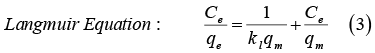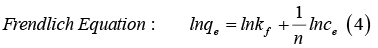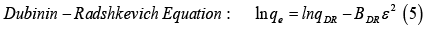where “qm” is the maximum sorption capacity (mg.g−1); “Kl” is the Langmuir sorption constant (dm3.mg−1) related to the energy of adsorption; “Kf (mol1-n.Ln.g−1)” and “n” are the Freundlich constants which indicate the adsorption capacity and the degree of dependence of sorption with equilibrium concentration, respectively. Moreover, qDR and BDR (mol2.J-2) in E-R equation are (D–R) constants which related to the adsorption capacity and energy, and ε is the Polanyi potential which is expressed by;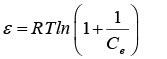(6)

where R is the gas universal constant (8.314 J.mol-1.K-1) and T is the absolute temperature (K). One of the important parameters of (D–R) equation which estimates the chemical or physical type of sorption process is E (kJ.mol-1). This parameter can be calculated according to the following equation: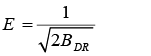(7)

The amount of obtained E from (D–R) equation can determine the type of sorption process. If 1< E < 8, a dominant mechanism is physical sorption. If 8<E<16, the chemical sorption will become a dominant mechanism. On the other hand, one of the significant parameters in Langmuir equation is the separation factor (RL) which indicates the type of isotherm to be favorable (0 < RL < 1) or unfavorable (RL > 1). This parameter can be calculated by the following equation;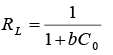(8)

Where C0 (mg/L) is the highest initial solute concentration. The RL indicates the type of isotherm to be favorable (0 < RL < 1) or unfavorable (RL > 1).

The value of all of the above mentioned constants is obtained from the slope and intercept of their linear plots of these equations (3-5). The linear plots of Langmuir, Freundlich and (D-R) equations were depicted in Figs. 5a to 5c, respectively. In addition, the calculated parameters were tabulated in Table 2.

As shown in Table 2, according to the highest correlation coefficients of the calculated linear regressions (R2), the Langmuir model is the most suitable model which fits the equilibrium adsorption data of fluoride ions on the adsorbents. This indicates the homogeneous distribution of active sites on these adsorbents. The calculated maximum adsorption capacity (qmax) of fluoride by these models shows that Al2O3-60-ZrO2 has high affinity toward fluoride ions (38.79 mg.g-1). It implies that the prepared hybrid materials increase the adsorption of fluoride in comparison with their pristine material, Al2O3-60, Al2O3-90, and ZrO2, (28.089, 20.161, 15.55 mg.g-1 ). Moreover, the calculated values of RL for all of adsorbents (0 < RL < 1) indicate that adsorption is favorable. On the other hand, the value of the obtained E (1< E < 8) shows that a dominant mechanism in adsorption process is the chemical sorption.

Effect of pH

Because of the variation of ion species with the change of solution pH, investigation of the effect of hydronium ions on the ion adsorption is one of the other significant and dominant parameters. Fig. 8 shows the diagram of the effect of solution pH on the percentage of fluoride ion onto these adsorbents. As shown, the adsorption rate of fluoride ions on Al2O3-60-ZrO2, Al2O3-90-ZrO2 and ZrO2 were almost independent on pH value within the range 3 to 8 while the adsorption rate of fluoride ions on Al2O3-60 and Al2O3-90 was highly dependent on pH value. According to hydrofluoric acid dissociation constant (6.8.10−4 at 25 °C), fluoride species are present as HF and F¯ at different pH values . At low pH, where the concentration of hydronium ions is high, protonation of the sorbent’s surface occurs. With the generation of positively charged sites, the electrostatic attraction force between these active sites and negative fluoride ions is increased and gives raised to the increase of the adsorption percentage of fluoride ions. At high pH, where the hydroxide ion concentration is high, adsorbent surface becomes negatively charged and increases the repellent force between the adsorbent´s surface and negatively fluoride ions. Thus, the decrease in fluoride adsorption percentage was observed.

Effect of nitrate and sulfate ions on the fluoride adsorption

The effects of coexisting anions such as nitrate and sulfate anions onto fluoride adsorption were investigated. The obtained results are represented in Figs. 9 and 10. As shown in Fig. 9, the adsorption percentage of fluoride for all of the adsorbents were independent of concentration nitrate ions for all of its concentration (0.001-1 M) while the considerable decrease of fluoride adsorption percentage was observed in all of adsorbents when sulfate ion was increased up to 0.1 M (Fig. 10). This is due to the affinity of these adsorbents toward sulfate ions and consequently, the competition between sulfate and fluoride ions toward the active site of adsorbents.

Effect of contact time

By using two common semiempirical kinetic models “the pseudo-first-order and pseudo-second-order equations” which are based on adsorption equilibrium capacity, the adsorption rate of ions on the adsorbents is studied. Therefore, these two models were used to simulate the kinetic adsorption data of fluoride on all of prepared adsorbents. In addition, linear arrangement of these equations is commonly used to investigate the validity of these models and to obtain their parameters. In this regard, the integrated and linearized forms of pseudo-first-order and pseudo-second-order are given as following equations 9 and 10, respectively: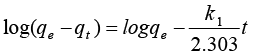(9)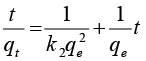(10)

Where qe and qt are the amounts of adsorbed fluoride ion concentration at equilibrium time and time t in mg.g-1k1 and k2 are the pseudo-first-order and pseudo-second-order constants expressed as min-1and g.mg-1.min-1, respectively.

Figs. 12a and 12b show the linear plots of log(qe−qt) versus t and t/qt versus t for all of the adsorbents. From the slope and intercept of these linear plots, parameters like K2, K1, and qe were obtained. These data accompany the amount of the calculated correlation coefficient (R2) were listed in Table 3. By comparing the data of correlation coefficient of linear regression in Table 3, it indicates that the pseudo-second-order model is suitable model to describe the kinetic profile. And that is because of the good fitness of this model with the kinetic data. A similar phenomenon has also been seen in the adsorption of fluoride on other adsorbents [34, 41]. In other words, according to the higher correlation coefficient of linear regression, the interaction between the solute and adsorbents is the most probable limiting step for the sorption process. Moreover, higher value of K2 Al2O3-60-ZrO2 and Al2O3-90-ZrO2 in comparison with three other adsorbents (Al2O3-60, Al2O3-90, and ZrO2) indicates that the adsorption process achieves equilibration very quickly. This quickly equilibrium time confirms the ability of the synthesized hybrid material for application of them in the removal of fluoride from aqueous solution.

To evaluate thermodynamic criteria and in order to determine which process will occur spontaneously, both Gibbs free energy and entropy factors must be calculated. Therefore, the effect of temperature on the adsorption behavior of fluoride ions has been studied in the range of 298- 328K. These thermodynamic parameters can be obtained by the following equations (11-12);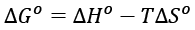(11)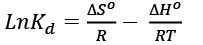(12)

Where the ΔG° is the change in Gibbs free energy in j mol-1ΔS° is the change in the entropy in jmol-1 and ΔH° is thechange in the enthalpy in j mol-1ΔS° is the change in the entropy in j mol-1T is the temperature in K, R is the universal gas constant (8.314 j.mol-1.K) and Kd is the distribution coefficient of elements which is calculated from the following formula;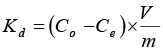(13)

Where CoV and Ce are the concentration (ppm) of fluoride ions in the solution phase before and after the adsorption process, respectively, V is the volume of initial solution in mL, and m is the dry mass of the adsorbent in g. By drawing the linear plots of lnkd vs. 1/T for all of the adsorbents and acquiring the slop and intercept of these linear graphs, the amount of ΔH° and ΔS° were obtained. Fig. 13 shows these linear graphs. The number of calculated parameters and the correlation coefficient of the linear regressions (R2) are listed in Table 4.

CONCLUSION

CONFLICTS OF INTEREST

There are no conflicts to declare.

#### References

2. Edition F. Guidelines for drinking-water quality. WHO chronicle. 2011;38(4):104-8.
8. NEELAKSHI VERMA HV, R. C. MEENA. REMOVAL OF FLUORIDE FROM DRINKING WATER: AN OVERVIEW INTERNATIONAL JOURNAL OF PHARMACEUTICAL RESEARCH AND BIO-SCIENCE. 2014;3(1):328-51.
9. Habuda-Stanić M, Ravančić EM, Flanagan A. A Review on Adsorption of Fluoride from Aqueous Solution. Materials. 2014;7(9).
11. Shimelis B, Zewge F, Chandravanshi BS. Removal of excess fluoride from water by aluminum hydroxide. Bulletin of the Chemical Society of Ethiopia. 2006;20(1):17-34.
16. Emmanuel K, Ramaraju K, Rambabu G, Rao AV. Removal of fluoride from drinking water with activated carbons prepared from HNO3 activation—A comparative study. Rasayan J Chem. 2008;1(4):802-18.
23. Goswami S, Dey S, Ghosh U. Studies on removal of fluoride by hydrated zirconium oxide. Chem Environ Res. 2004;13:117.
24. Sajidu S, Kayira C, Masamba W, Mwatseteza J. Defluoridation of groundwater using raw bauxite: rural domestic defluoridation technology. Environment and Natural Resources Research. 2012;2(3):1.
26. Waghmare SS, Arfin T. Fluoride removal from water by aluminium based adsorption: A review. Journal of Biological and Chemical chronicles. 2015;1(1):1-11.
38. Adak MK, Mondal B, Dhak P, Sen S, Dhak D. A comparative study on fluoride removal capacity from drinking water by adsorption using nano-sized alumina and zirconia modified alumina prepared by chemical route. Advances in Water Science and Technology. 2017;4(01):01-10.
39. Sing KS. Reporting physisorption data for gas/solid systems with special reference to the determination of surface area and porosity (Recommendations 1984). Pure and applied chemistry. 1985;57(4):603-19.